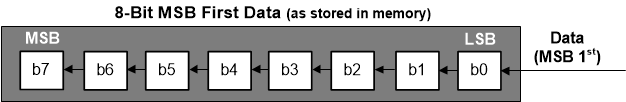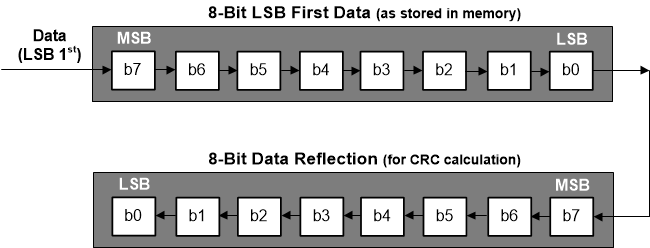SBAA106A June   2020  – August 2021

2. 1Introduction
3. 2Simple Checksum
4. 3CRC
1. 3.1 CRC Generic Computations
1. 3.1.1 Using XOR Bitwise Computation
2. 3.1.2 Using Lookup Tables
3. 3.1.3 CRC Computation Differences Between the ADS122U04 and ADS122C04
5. 4Hamming Code
1. 4.1 Hamming Code Computation
1. 4.1.1 Hamming Code Computation Example
2. 4.1.2 Validation of Transmitted Data
6. 5Summary
7. 6References
8. 7Revision History

### 3.1.3 CRC Computation Differences Between the ADS122U04 and ADS122C04

As was mentioned in the overview, the CRC value is computed relative to the bit order of the data transmission as it is shifted out of the device. The data transmission from the "U" devices are much different as compared to the "C" devices. The "U" devices use UART transmission with data transmitted LSB first whereas "C" devices use I2C transmission with data transmitted MSB first. The MSB first data are read into the microcontroller peripheral and reassembled in the same manner as the data are transmitted (when using Big Endian format) as shown in Figure 3-5.Figure 3-5 Contents of Memory for Shifting Data In MSB 1st

The CRC computation for the "U" devices follow the same process as shown in Figure 3-3. However, the difference for the "U" device is the shift register starts with the LSB first instead of the MSB. The outcome directly impacts the CRC computation and the byte order in which the data are stored into memory.

When computing the CRC by a code function it is helpful to understand the method by which data are stored. As an example, consider a memory made up of byte (8-bit) addressable locations. A 32-bit integer is made up of four adjacent bytes. Consider the integer as an array of four bytes. If the value stored in the first element of the array is the most significant byte (MSB) of the integer, the value is stored as Big Endian. If the least significant byte (LSB) is stored as the first element of the array, then the integer is stored as Little Endian. Table 3-1 shows a comparison of how the 32-bit value is stored in memory. When data are transmitted MSB first, the order of transmission follows the Big Endian format. However when data are transmitted as LSB first, the order of transmission follows the Little Endian format. For the purposes of this discussion the Big Endian format will be used by the microcontroller. However, in both methods the most significant bit of the byte array element is bit 7 and the least significant bit is bit 0.

Table 3-1 Memory Addressing for 32-bit Data
32-bit DataMemory AddressBig EndianLittle Endian
0A0B0C0Dh0h0Ah (MSB)0Dh (LSB)
1h0Bh0Ch
2h0Ch0Bh
3h0Dh (LSB)0Ah (MSB)

Computation of the CRC is based simply on the data for the bit and byte order it was transmitted. However, the LSB first data is transferred to the microcontroller UART peripheral where the interface will shift the data LSB first, but the contents in memory will be in the reverse order that it was transmitted. As the transmitted data are in reverse order of the data in memory, the computed value of the CRC by the microcontroller will be incorrect in both bit and byte order. For LSB first transmitted data, both the byte order and the bit order within each byte must be reversed, or reflected, for the computation (see Figure 3-6).Figure 3-6 Contents of Memory for Shifting Data In LSB 1st Requiring Reflection for CRC Computation

If the microcontroller memory is Big Endian and the UART conversion data received is stored as a signed 32-bit integer, it is not enough to simply reverse the order of the bytes representing the integer value. Part of the reason is the original data are 24-bit (16-bit for 16-bit devices) and the integer value is sign-extended. In addition, most microcontroller UART peripherals will clock the data into an 8-bit shift register LSB first, but store the byte in the opposite orientation in memory. The peripheral will realign the bit order in memory that is the reverse, or the reflection, of the order of bits transmitted. However, when computing the CRC for the data packet the order and number of bits must match the same order and number of bits transmitted. For example, if a 24-bit conversion result is stored to a signed 32-bit value, then the CRC computation must take place using the original binary two's complement 3 bytes of data with the proper reflection as opposed to a 4 byte signed number that has been sign-extended.

As an example of the steps required, an array (cData) representing 24-bit conversion data is created from an array (sData) for a signed 32-bit integer. The new array (cData) is used to compute the CRC for comparison to the CRC transmitted from the ADS122U04.

1. Copy the sData array to the cData array where the cData array represents the Little Endian format as illustrated in Figure 3-7.
2. As the conversion data is 24-bit, do not use the sign-extended byte in the cData array.
3. The cData array now contains the correct byte order, but not in the correct bit order.
4. For each byte element in the cData array, reflect the order of bits so that the bit order is the same as originally transmitted as shown in Table 3-2.
5. Compute the CRC for the first three elements in the cData array.Figure 3-7 Changing Byte Order to Reflect LSB 1st
Table 3-2 Reflection of Signed 32-bit Integer to 24-bit Using LSB 1st for CRC Computation
Array ElementsData (32-bit)cData (24-bit)cData Reflected
000h78h (0111 1000b)1Eh (0001 1110b)
14Eh68h (0110 1000b)16h (0001 0110b)
268h4Eh (0100 1110b)72h (0111 0010b)
378hN/AN/A